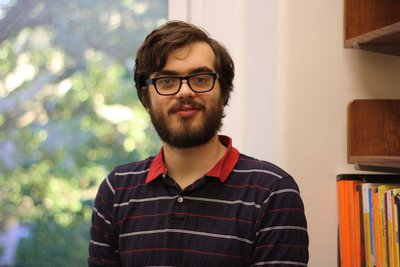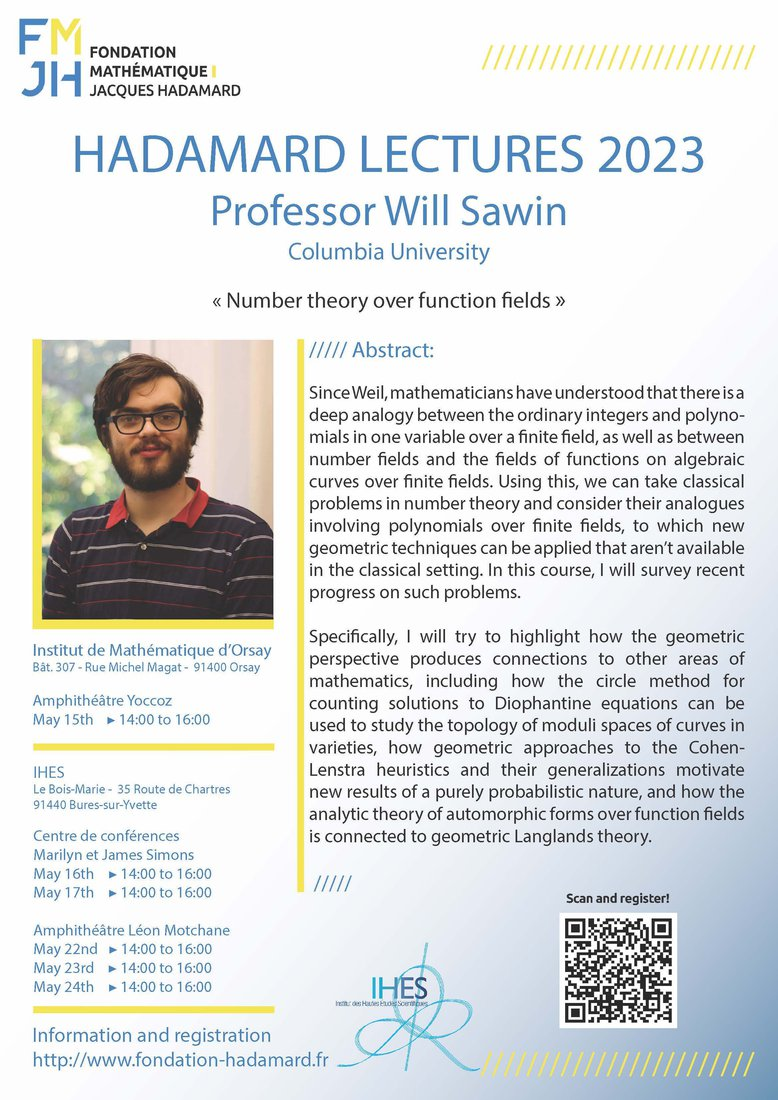# Will Sawin

### (Université Columbia à New York)

Lauréat du prix SASTRA Ramanujan 2021
dont les recherches portent entre autres sur les applications
de la cohomologie étale à la théorie analytique des nombres.

## 15 mai 2023 à l'Institut de Mathématique d'Orsay 16, 17, 22, 23 et 24 mai 2023 à l'IHES

### "Number theory over function fields"

Abstract: Since Weil, mathematicians have understood that there is a deep analogy between the ordinary integers and polynomials in one variable over a finite field, as well as between number fields and the fields of functions on algebraic curves over finite fields. Using this, we can take classical problems in number theory and consider their analogues involving polynomials over finite fields, to which new geometric techniques can be applied that aren't available in the classical setting. In this course, I will survey recent progress on such problems.

Specifically, I will try to highlight how the geometric perspective produces connections to other areas of mathematics, including how the circle method for counting solutions to Diophantine equations can be used to study the topology of moduli spaces of curves in varieties, how geometric approaches to the Cohen-Lenstra heuristics and their generalizations motivate new results of a purely probabilistic nature, and how the analytic theory of automorphic forms over function fields is connected to geometric Langlands theory.